Courses
Courses for Kids
Free study material
Offline Centres
More

# Circle Corner and SidesLast updated date: 28th Nov 2023
Total views: 97.2k
Views today: 1.97k## Introduction to Corner and Sides of Plane Figures and Circles

The majority of the things we come up with may be categorised by simple shapes. A closed, two-dimensional, or flat form is referred to as a plane shape. Different plane shapes have different properties, such as different numbers of sides or corners. Where two sides converge is referred to as a corner or vertex. An element of geometry known as a side is a straight line.

In this article, we will briefly discuss the circle and the corners and sides of plane figures. Squares, triangles, and circles are examples of two-dimensional shapes known as planes. Sides are the straight lines that make up a plane form. Corners are the places where two sides converge.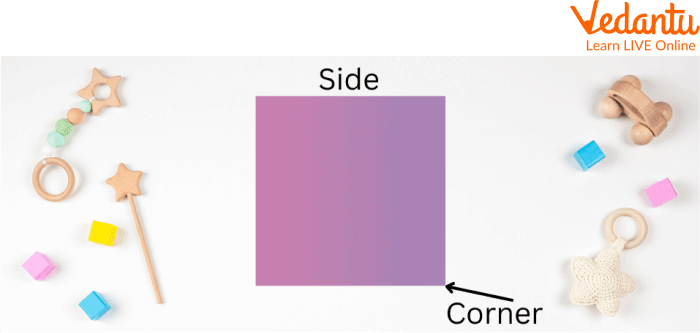Corner and Sides of Plane Shapes

## What are Plane Shapes?

A shape can be defined as the boundary or outline of an object. A plane shape is a two-dimensional closed figure that has no thickness. A plane in geometry is a flat surface that extends into infinity in all directions. It has infinite width and length, zero thickness, and zero curvature.

## Various 2D Shapes

Look at a piece of paper. Its length and width are visible. These are the length and width, sometimes known as the two dimensions. There is no depth in a shape that is only two dimensions. When straight or curved lines are connected, a closed shape known as a 2D shape is produced.

1. Square: Squares are four-sided quadrangles with four equal sides and four right angles.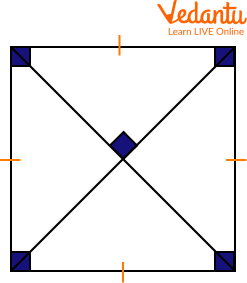Square

Number of Sides = 4

Number of corners = 4

2. Rectangle: A rectangle is a quadrilateral having four right angles and two sets of equal and parallel opposite sides.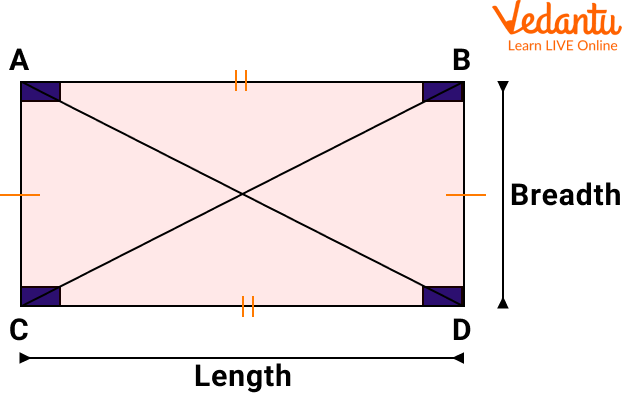Rectangle

Number of Sides = 4

Number of corners = 4

3. Triangle: A closed triangle has three sides, three angles, and three vertices.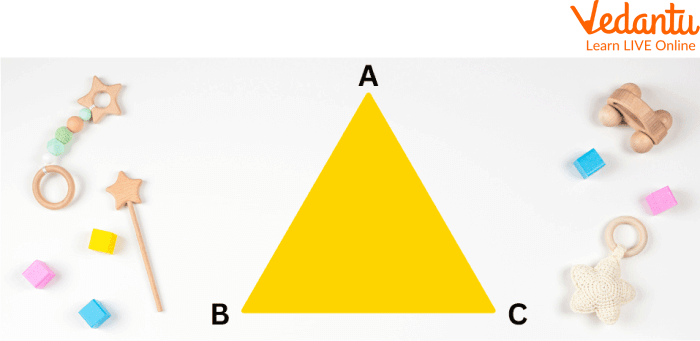Triangle

Number of Sides = 3

Number of corners = 3

4. Oval: The shape of an oval lacks a straight line, a corner, or a side. It has curving lines that form a closed shape.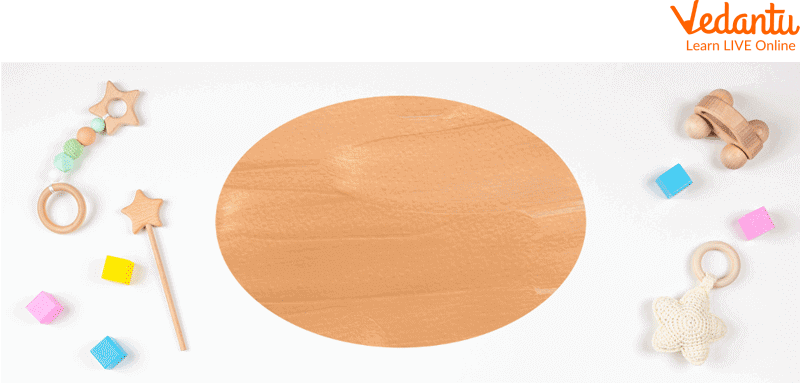Oval

Number of Sides = 0

Number of corners = 0

## What is a Circle?

A circle is a two-dimensional object made up of points that are spaced out from a given point (centre) on the plane by a fixed or constant distance (radius). The fixed point is referred to as the circle's origin or centre, and the fixed distance between each point and the origin is referred to as the radius. Let's learn more about how many sides are there in a circle.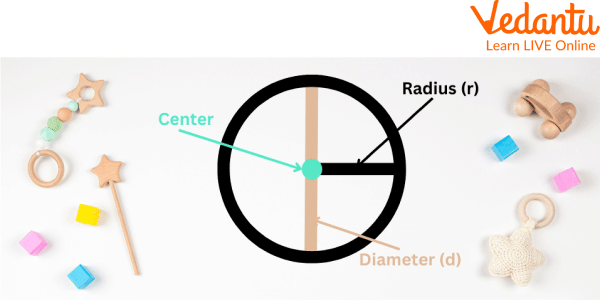Circle Corner and Sides

## How many Sides are there in a Circle?

A line segment between two locations is referred to as a side. It is impossible to fit a line between the points of a circle because there are infinitely many points which correspond to infinitely many sides. As a result, a circle would technically have no sides. There are no corners and sides in a circle.

## Solved Examples

Q1. Help Tanu count the number of sides in the given shape. Is the shape closed or open?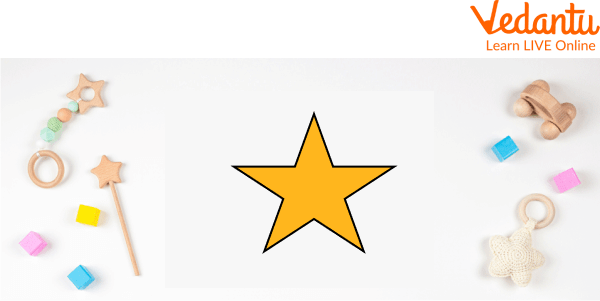Star

Ans: Count the number of straight lines in the star. There are 10 straight lines.

We can trace the outline of this star without any break. Hence, it is a closed shape.

It is a closed shape with 10 sides and 10 corners.

Q2. What shapes have 4 sides and 4 corners?

Ans: Any quadrilateral will have 4 sides and 4 corners. For example: Squares and Rectangles are shapes that have 4 sides and 4 corners.

Q3. A circle is a polygon with infinitely many sides. Determine whether the following statement is true or false.

Ans: A polygon is a closed-plane figure that has three or more straight sides while a circle is a closed-plane figure with no straight sides. As a result, a circle is not a polygon with infinitely many sides. Hence, the given statement is false.

## Practice Problems

Q1. How many sides and corners are there in the octagon?

Ans: 8

Q2. Name the figure with five sides and five corners.

Ans: Pentagon

Q3. Name the shape having infinitely many sides.

### Summary

Throughout this article, we have discussed the plane figures and circle corners and sides. A circle is defined as a spherical shape without edges or corners. It is a shape formed by a curved line. It's circular, and each point on the curved line is an equal distance from the centre. We also covered various 2D shapes such as squares, rectangles, etc. This article also explains how many sides are there in a circle. We also learn what shapes have 4 sides and 4 corners. We have provided some solved examples and practice problems based on plane figures and circles to boost your concept of plane figures and circles.

## FAQs on Circle Corner and Sides

1. Is the shape of a sphere solid or plane?

A solid figure is three-dimensional while a plane figure is only two, making the sphere a solid figure. A solid figure has length, width, and depth, while a plane figure only has length and width. A plane can be drawn on using many substances, such as a blackboard, paper, etc. On a plane, a solid figure cannot be drawn. For instance, the shapes for a plane figure are a circle, triangle, and so on.

2. When depth is added to a 2D shape it becomes?

When depth is added to a 2D shape with length and width, it becomes a 3D shape.

3. What characteristics do circles have?

The following are a few characteristics of circles:

1. A closed 2D form that isn't a polygon is a circle. It has one face that curves.

2. If two circles have the same radius, they are said to be congruent.

3. Equal chords are always located equally from the circle's centre.

4. The centre of the circle is the perpendicular bisector of a chord.

5. The tangents drawn at the diameter's endpoints are perpendicular to one another.